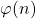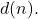# On certain equations and inequalities involving the arithmetical functions φ(n) and d(n) – II

József Sándor
Notes on Number Theory and Discrete Mathematics
Print ISSN 1310–5132, Online ISSN 2367–8275
Volume 29, 2023, Number 1, Pages 130–136
DOI: 10.7546/nntdm.2023.29.1.130-136

## Details

### Authors and affiliations

József SándorDepartment of Mathematics, Babeș-Bolyai University
Cluj-Napoca, Romania

### Abstract

In papers  and  we have studied certain equations and inequalities involving the arithmetical functionsandIn this paper we will consider some other equations. Some open problems will be stated, too.

### Keywords

• Arithmetic functions
• Equations
• Inequalities

• 11A25

### References

1. Guy, R. K. (2004). Unsolved Problems in Number Theory (3rd ed.). Springer-Verlag.
2. Sándor, J., Mitrinović, D. S., & Crstici, B. (2005). Handbook of Number Theory I. Springer.
3. Sándor, J. (2020). On the equation on φ(n) + d(n) = n and related inequalities. Notes on Number Theory and Discrete Mathematics, 26(3), 1–4.
4. Sándor, J., & Atanassov, K. T. (2021). Arithmetic Functions. Nova Science Publ., New York.
5. Sándor, J., & Bhattacharjee, S. (2022). On certain equations and inequalities involving the arithmetical functions φ(n) and d(n). Notes on Number Theory and Discrete Mathematics, 28(2), 376–379.

### Manuscript history

• Revised: 17 February 2023
• Accepted: 2 March 2023
• Online First: 7 March 2023Ⓒ 2023 by the Author.